The Response Spectrum analysis (RS analysis) allows getting the maximum value of forces, displacements and base reactions of a structure, starting from a design spectrum defined in terms of period (T) vs. pseudo-accelerations (Sa). The design spectrum is usually given by design codes.

The equation of motion involves the mass matrix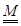, the stiffness matrix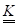and the damping matrix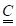.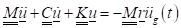(1)

In Eq. 1,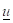is the displacement vector we want to find as solution of the system,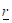is the dragging vector, which specifies for which spatial directions the external ground acceleration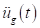is applied.

The base for the space of all the possible configurations can be described by linearly combining the eigenvectors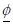that can be extracted from the system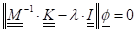(2)

being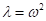the square of the cyclic frequency for each mode, and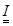the identity matrix.

Hence, the displacement can be written in terms of the product between the matrix collecting all the normalized eigenvectors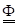and the modal coordinates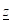.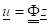(3)

By using Eq. 3 in Eq. 1, we get: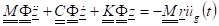(4)

By multiplying both members by ΦT we get: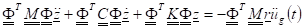(5)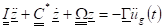(6)

Where  C* is the modal damping matrix, Ω  is called spectral matrix and Γ  is the vector containing the participation factors.

The mass excited by the motion is: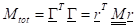(7)

The participation factor is the ratio of the excited mass over the total mass of the system: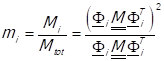(8)

The mass excited in the i-th mode is: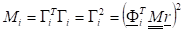(9)

Then, the percentage of the mass in Eq. 9 would be: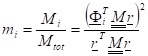(10)